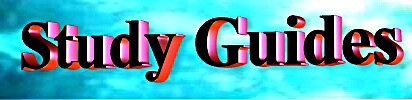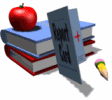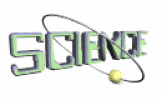On-Line Practice Tests (1) Type in your name at the top of the "form" (2) Take the "test" (3) Click on "Check Your Work" at the bottom and the practice test will be automatically scored.   (4) Take the test as many times as  you like for practice. (5) Your scores are NOT emailed to the teacher, so you are free to use the on-line test as  a study guide and take it as many times as you need to in order to master the material. Can I Look at or Download Items Ahead of Time? Yes, if you would like to work ahead, it is perfectly OK to look at or download items before we cover those subjects in class.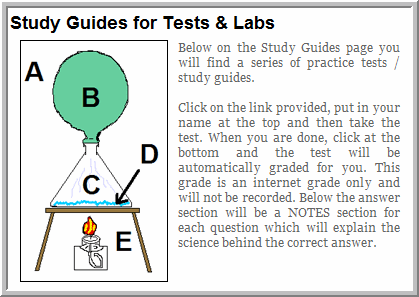Physics Overview

-- How to Study Physics
-- Word Problem Template
 Labs Activities Formulas* & Concepts Bottle Rocket Lab Inclined Plane Lab Levers Lab Pulleys Lab Friction Lab Ball Drop Lab #1 Ball Drop Lab #2 Low Friction Cart Lab Projectile Lab Collision Tracks Gyroscopes Toys in Space (video) Cartoon Physics (clips) CD Glider Hoop Dreams Speed & Velocity* Acceleration* Force* Momentum* Work* Power* Potential Energy* Kinetic Energy* Efficiency* ======================= Gravity Balanced & unbalanced       Forces Newton's 1st Law Newton's 2nd Law Newton's 3rd Law Circular Motion Mechanical Advantage

Physics Chapter 11 -- Motion (Speed & Acceleration)

LEARN

 BrainPop - Animations (Login: hammondschool -- Password: skyhawks) Web Page Library Acceleration Distance, Rate, & Time Speed Average Speed Position-Time Graph Velocity of a Line Average Acceleration
 Read -- Holt Physical Science Quiz Questions 1. pp. 363 - 369             (speed) 2. pp. 370 - 371           (graphs) 3. pp. 372 - 379    (acceleration) 4. pp. 380 - 382             (forces) 5. pp. 382 - 387            (friction) 6. p. 389                 (Summary) 7. pp. 363 - 421 (All Questions) 1.  Speed 2.  Graphing Motion 3.  Acceleration 4.  Forces 5.  Friction 6.  Summary 7.  Quiz Questions (#1 - #6)

PRACTICE

Study Guides for Chapter 11 -- Motion (Speed & Acceleration)
- Formula Practice
(word problems for each formula -- you solve them)
- Units of Measurement Practice
(you match them up with formulas)
- Vocabulary Practice (you find the definitions)
- Picture Problems (you figure out the solutions)

Flash Card Sets for Chapter 11 -- Motion (Speed & Acceleration)

- Paper Flash Cards
- Internet Flash Cards (Quiz Questions #1 - #6 + answers )
- Picture Problems
(sample problems  to consider -- with solutions)
- Sample Word Problems + Solutions (Roll cursor over answer box for solution)

TEST YOURSELF

Physics Chapter #11 -- Practice Tests: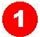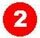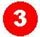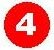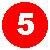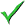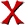Chapter #11 (multiple choice) Chapter #11 (completion) Chapter 11 mixed word problems with explanations coming soon coming soon
 (1) Click on a  test number. (2) Type in your name at the top. (3) Take the test. (4) Click on "Check Your Work" at the bottom and the practice test will be automatically scored.  Take the test as many times as  you like for practice.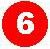Speed Problems Acceleration Problems Momentum Problems

web page

Physics Chapter 12 -- Forces (Newton's Laws & Momentum)

LEARN

 BrainPop - Animations (Login: hammondschool -- Password: skyhawks) Web Page Library Newton's Laws of Motion Gravity Isaac Newton Inertia Centripetal Force Variables Newton's 2nd Law Newton's 3rd Law Projectile Motion Weight Momentum How Can Anything Move? Net Force What is a Force?
 Read -- Holt Physical Science Quiz Questions 1. pp. 397 - 399          (1st Law) 2. pp. 400 - 402         (2nd Law) 3. pp. 403 - 406            (gravity) 4. pp. 407 - 411           (free fall) 5. pp. 412 - 413          (3rd Law) 6. pp. 414 - 418     (momentum) 7. p. 389                 (Summary) 8. pp. 347 - 389  (All Questions) 1. 1st Law 2. 2nd Law 3. Gravity 4. Free Fall 5. 3rd Law 6. Momentum 7. Summary 8. Quiz Questions (#1 - #7)

PRACTICE

Study Guides for Chapter 12 -- Newton's Laws of Motion
- Formula Practice
(word problems for each formula -- you solve them)
- Units of Measurement Practice
(you match up with formulas)
- Vocabulary Practice (you find the definitions)
- Picture Problems
(you figure out the solutions)
- Study Guide & Vocabulary Lists for Chapter 12  (overview)

Flash Card Sets for Chapter 12 -- Newton's Laws of Motion

- Paper Flash Cards
- Internet Flash Cards (Quiz Questions #1 - #7 + answers )
- Old Flash Cards on Newton's Laws
(2005)
- Picture Problems
(sample problems  to consider -- with solutions)
- Sample Word Problems + Solutions (Roll cursor over answer box for solution)

TEST YOURSELF

Physics Chapter #12 -- Practice Tests:Chapter #12 Concepts (Multiple Choice) Identifying Vectors  of Force Identifying Examples  of Newton's Laws Physics  Puzzlers: Figuring out WHAT HAPPENS & WHY according to Newton's Laws of Motion What's happening in these pictures? Can you identify concepts, motion, correct Law?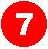Chapter #12 Concepts (Completion) Coming Soon Chapter #12 Formulas with explanations

Physics Chapter 13 -- Work, Energy, & Simple Machines

LEARN

 BrainPop - Animations (Login: hammondschool -- Password: skyhawks) Web Page Library Kinetic Energy Potential Energy Power Work Inclined Plane Levers Wheel & Axle Energy Kinds of Energy Net Force Work Levers #1 , #2 ,  Quiz Simple Machines Roller Coasters Pulleys
 Read -- Holt Physical Science Quiz Questions (77) 1. pp. 431 - 432                       (work) 2. pp. 433 - 434                     (power) 3. pp. 434 - 437                        (MA) 4. pp. 438 - 443     (simple machines) 5. pp. 444 - 452                    (energy) 6. pp. 453 - 457            (conservation) 7. pp. 459 - 461                (efficiency) 8. p. 465                          (Summary) 9. pp. 429 - 465          (All Questions) 1. Work 2. Power 3. MA 4. Simple Machines 5. Energy 6. Conservation of Energy 7. Efficiency 8. Summary 9. Quiz Questions (#1 - #8)

PRACTICE

Study Guides for Chapter 13 -- Work, Energy, & Simple Machines
- Formula Practice
(one word problem for each of the 7 formulas -- you solve them)
- Units of Measurement Practice
(8 units listed -- you match up with formulas)
- Vocabulary Practice (13 words listed -- you find the definitions)
Picture Problems (you figure out the solutions)

Flash Card Sets for Chapter 13 -- Work, Energy, & Simple Machines

- Paper Flash Cards
- Internet Flash Cards (Quiz Questions #1 - #8 + answers )
- Identify Levers Flash Cards
(10 pictures - you identify which class of lever)
- Picture Problems
(sample problems  to consider -- with solutions)
- Sample Word Problems + Solutions (Roll cursor over answer box for solution)

TEST YOURSELF

Physics Chapter #13 -- Practice Tests:Chapter #13 44 Concepts   (multiple choice) Chapter #13 22 Word Problems with explanations Chapter 13 66 Concepts (Completion)

Physics Final Exam

Concepts

Vocabulary

Mixed Word Problems

Work & Energy
Vectors

Work & Energy
- Ch13
Forces - Ch 12
Motion - Ch 11

Lab Review

Picture Problems

All Formulas

Bottle Rockets
Inclined Plane
Levers
Ball Drop #1
Ball Drop #2
Low Friction Cart

Formula Review

(11 formulas)

 Multi-Media Unit (Mr. C's favorite low-cost or free applications) OpenOffice.org (Version 3.1) (free) This  link will take you to the web page for OpenOffice.org. We are now  using this as an Open Source alternative to Microsoft Word, Excel, and POWERPOINT. This software is OPEN SOURCE -- meaning that it is FREE to the public -- no charge. The word processor will read Microsoft Word files and the presentation package, called "IMPRESS," will read Microsoft PowerPoint files. All of the computers in the 7th grade HAG science classroom are now  running OpenOffice.org software. We are no longer using Microsoft Office for word processing or for 'Powerpoint' style presentations. . .exe format PowerPoint Viewer 2007 (free) Hyperstudio v. 5 (\$89.95 Mac OS & Windows) Audacity -- Free, Cross-Platform Sound Editor (free) Acoustica MP3 Audio Mixer (\$24.95) This multi-track audio program lets you mix, cross-fade, and overdub audio files. A must for podcasting!